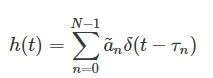## Creating Frequency Selective time invariant channelStarted by 6 years ago2 replieslatest reply 6 years ago188 views

Hi everyone

I am wondering if anyone can explain how to generate frequency selective time invariant channel. This means that no Doppler shift and I only need to implement multipath Rayleigh fading channel. I know it is a FIR filter and can be implemented as Tapped delay line. However I do not understand since the equation can be written asand how can I implement this.like how to shift delta function.Is this equation implemented like with for loop and each time instant channel gain is multiplied by a shifted delta function?

Thanks.

[ - ]"The equation" that you refer to is an impulse response.  You might want to study up on what that means.  If you did know what it means, you'd know that it defines the system

$h \left(x\left(t\right)\right) = \sum_{n=0}^{N-1} \tilde a_n x \left( t - \tau_n \right)$

where $$x\left(t\right)$$ is the input signal and the output signal is implied.

[ - ]